Study Guide

# Electricity and Magnetism Terms

## Electricity and Magnetism Terms

### Ampère's Law

The magnetic field inside an imaginary Ampèrian loop—drawn by you, of course—is proportional to the current flowing through the loop. This is given by the formula Bl = μ 0I, where l represents the total length of the section(s) of the loop parallel to the magnetic field lines. The loop itself can be a rectangle or a circle (or any other shape you want, but good luck using the asymmetric octagonal loop you've drawn).

### Capacitance

Capacitance (C) is the relationship between the amount charge on two objects and the voltage that appears between them, given by the formula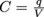.(In other words, capacitance is a measure of the capacity of an object to store charge.) Its SI unit is the farad (F), named after Michael Faraday, which is equal to one coulomb divided by one volt.

One farad is a gigantic unit—typical capacitors can range from the pico- (10-12) to milli- (10-3) farad range. Capacitance is purely a product of geometry, and can be altered by changing the size of the objects or the distance between them.

### Capacitor

Capacitors are used in all sorts of electric circuits as a quick way to store charge and then discharge it—the flash in a cheap disposable camera, for example, is just a big capacitor and a light bulb. The basic kind of capacitor is a parallel plate capacitor, which stores charge between two square plates, and has a capacitance of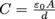.Other configurations of capacitors have different capacitances, given by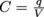.The energy stored in any capacitor is given by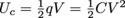(which can be very large for big capacitors charged with decent voltages—a big cap can do some serious crunchatizing).

### Charge

Charge is the basic unit of electricity, abbreviated q—since anything sensible would be silly—and measured in coulombs (C), named after Charles-Augustin de Coulomb, an eighteenth century physicist who spent a lot of time playing with charged spheres. Charge can be used to describe the size of the force an object feels when it is exposed to other charged objects, and can either have positive or negative polarity.

Charge is an intrinsic property of matter, the same way mass is; however, unlike mass, charge comes in nice discrete packages. Static electricity represents a successful buildup of charge, Pickett's Charge does not.

### Circuit

A circuit is a collection of conducting wire and various electrical elements that constrains electron flow, stopping the little buggers from flying around all nimbly-bimbly in an effort to get them to move out and accomplish something useful with their lives instead of living off of mom and dad's increasingly strained largess. Circuits are present in every electronic device on the market, and can be as simple as a battery, two wires, and a light bulb, or as complex as modern computer motherboards.

### Conductor

A material that conducts heat or electricity, which means electrons can easily flow through the object.

### Conservation of Charge

As an intrinsic property of matter, charge must be conserved, in the same way mass or energy or momentum are conserved. Charge cannot be created or destroyed; it can't even be turned into other forms. It's always present, but can be canceled out or enhanced by opposite or similar charges, respectively. So keep on trying to save the rainforests—charge will be just fine without you.

### Coulomb's Law

Explains the electrostatic interaction between charged particles. If the two charges have the same sign, the electrostatic force between them is repulsive. If they have different signs, the force between them is attractive. Charges close together mean stronger bonds, while charges far apart mean weaker bonds. It ain't fiction just a natural fact.

### Current

The movement of charge is called current, abbreviated I. Current can be calculated by watching how much charge passes a point in a specific length of time: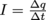. Its SI unit is the ampere (A)—or just the amp—named after André-Marie Ampère (of Ampère's Law fame). One amp is equal to one coulomb per second of charge flow, but since a coulomb is very large (one electron's charge is 1.6 × 10-19 C), 1 A is a decent amount of current.

Because current is a measure of the change of positive charge, the direction of current flow is opposite the direction of electron flow. You can thank Ben Franklin for screwing that one up for everybody—apparently he was too busy founding a country and wooing French women to think about the convention for electricity he was also inventing. You know, in his spare time.

### Electric Field

Charged particles exert forces on other charged particles without touching them—the force is transferred through the invisible electric field (E) that all charged particles create, just like Earth's gravitational field still pulls you down when you jump off the ground.

The electric field of a single charged particle at a distance r away from it is given by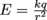, which means Coulomb's Law for the force one particle of charge q feels in the field of another charged particle can be rewritten as Fe = qE. Electric field is therefore expressed in units of newtons per coulomb.

The bigger the charge on a particle, the stronger the electric field it creates—if you charge it, particles will come...or be repelled, depending both on charge polarity and their feelings on Kevin Costner.

We represent fields by drawing field lines, a graphical tool for visualizing what's otherwise invisible. The stronger the field, the more lines you draw.

### Electric Potential (Voltage)

Since charges attract or repel each other, a specific configuration of charged particles must have some potential energy associated with it—it's possible to turn electric attraction/repulsion into kinetic energy, and we know energy must be conserved. This is called electric potential, but the more familiar term for it, usually used in the context of circuits, is voltage.

Electric potential (V) is measured in volts, named after Alessandro Volta, widely credited as the inventor of the modern battery. One volt is equal to a joule per coulomb—that is, the amount of energy it takes to move 1 C of charge to a specific location in the electric field of a second charge.

In formula form, the electric potential at a point r away from charge q1 is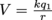The energy needed to move a charge q2 from an infinite distance away (where the potential is zero) to that location is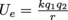.

Just like potential energy in mechanics, electric potential is a relative measurement. The potential difference between point a and point b can be found by using the formula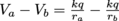. Circuits are all about celebrating differences.

### Electromagnetism

Electromagnetism is a combination of the disciplines of electricity and magnetism, which are inextricably intertwined—moving charges create magnetic fields, and moving magnetic fields can create voltages. Electromagnetism is responsible for countless inventions in the modern world, from televisions to cell phones to blenders to polarized sunglasses.

### Electromotive Force

The electromotive force, or emf (abbreviated with the very fancy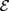;said aloud as "ee-em-eff"), is a highfalutin name for the voltage that pushes electrons around a circuit. This is usually divided into two groups: emfs generated within a battery, and motional emfs, or those that appear with changes of magnetic flux as given by Faraday's Law. Contrary to its name, an emf is a voltage (and is measured in volts)—not a force at all.

Faraday's Law of Electromagnetic Induction and Unnecessarily Long Names for Fundamental Phenomena states that any change in magnetic flux through a loop or coil of wire will create a voltage that appears across the wire, given by the formula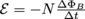.

### Flux

A field's flux (Φ) is defined as the amount of field lines passing through a given area perpendicular to the field, such as the area within a loop of wire. For a field X, the flux through an area A perpendicular to the field would be given by ΦX = XA. Flux is often more descriptive than just field strength at a point, as it lets us describe how fields interact with objects on a larger scale. Strong field, small loop of wire? Lots of flux. Weak field, big loop of wire? Still lots of flux.

### Gauss' Law

A charge q creates an amount of electric flux given by Gauss' Law: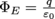.This is usually expanded and written as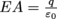,where A is the surface area of a Gaussian surface drawn around the charge, or around a collection of charges. Gauss was an inclusive guy.

### Inductance

Inductance (L) describes the size of the voltage created in an object when the current passing through the object changes. Objects with large inductances can see large voltages across them even with small changes in current, as given by the formula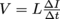.Inductance is measured in henries, named after Joseph Henry, who discovered electromagnetic inductance around the same time as Faraday—but missed the boat on publishing and so only got a corollary instead of a law.

### Inductor

An inductor is a circuit element that usually consists of a coil of wire, and is used to store energy in a magnetic field according to the formula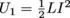.Inductors pop up in all sorts of useful circuits, from audio filters to loudspeakers to transformers (the power line kind, not the Optimus Prime kind).

### Insulator

Insulators prevent the free flow of electrons, and therefore do not let current flow through them. Most materials that aren't metal fall into this category, such as rubber or plastic. The insulator around a power cord is what lets you plug in your vacuum cleaner without getting all electric feeled.

### Kirchhoff's Current Law

The sum of all current entering a circuit node (the point where multiple paths in the circuit connect) must equal the sum of all current leaving the node. Two currents enter, one current with a magnitude equal to the sum of the first two currents leaves.

### Kirchhoff's Voltage Law

The net change in voltage around any complete loop (one that current can flow around) in a circuit must be zero. That means all the electric potential energy given to electrons zooming around the wires by a battery must be dissipated in resistors, stored in capacitors, etc. by the time the electrons make it back to the battery. Kirchhoff's Voltage Law is really just a restatement of the law of conservation of energy.

### Lenz's Law

Faraday may get credit for the whole law of electromagnetic induction, but it's Lenz who's responsible for the crucial minus sign in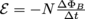. Lenz discovered a simple but fundamental property of electromagnetism: the voltage induced by a change in magnetic flux will always try to create a current that cancels out that change in flux. This is often summed up pithily as nature abhors change. (Except for, you know, autumn. And chameleons. And evolution. And sunrises. And...okay, so maybe nature just abhors changing magnetic fields.)

### Lorentz Force

A charged particle traveling in a magnetic field will experience a force dependent on its charge and its speed; this is called the Lorentz force and is given by Fb = qvBsin θ. This can be combined with Coulomb's Law to give a full equation for the net force on a charged particle in both electric and magnetic fields: F = Fe + Fb = qE + qvBsin θ. don't forget the full vector form, either: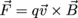.Lorentz basically figured out how to be Magneto.

### Magnetic Field

A magnetic field is a force field created out of electromagnetism. It's not quite what sci-fi fans imagine, but it'll have to do for now.

### Ohm's Law

The voltage drop across a resistor is a product of the current through the resistor and the resistor's resistance: V = IR. This is the pièce de résistance of solving circuit problems.

### Permeability of Free Space

The permeability of free space, or the magnetic constant, is the fundamental constant that describes the strength of a magnetic field's ability to penetrate a vacuum—or, for all intents and purposes (at least, our intents and purposes) air. The constant is given by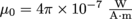. It is related to the permittivity of free space by the speed of light in vacuum,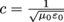, and has nothing to do with the kind of perm you'd get in a salon.

### Permittivity of Free Space

The permittivity of free space, or the electric constant, is the fundamental constant that describes the strength of an electric field's ability to penetrate a vacuum—or, for all intents and purposes (at least, our intents and purposes) air. The constant is given by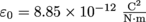. It is related to the permeability of free space by the speed of light in vacuum,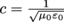, and has nothing to do with the kind of permission you'd get to take your dad's car for a spin.

Notice any parallels?

### Polarity

Both electric charges and magnet ends have polarity, which is a fundamental binary attribute that describes how the charge or the magnet interacts with other charges or magnets. Electric charge can be positive or negative; like charges repel and opposites attract. Magnets have both a north end and a south end; again, like ends repel one another and opposite ends attract.

### Quantization of Charge

The fundamental unit of charge is the magnitude of the charge of an electron, 1.6 × 10-19 C. Charge will always be present in multiples of the charge of an electron, or occasionally in multiples of one large bull. ¡Olé!

### Resistance

Resistance (or La Résistance) is the degree to which a material can prevent current (or bad guys) from moving through it. It is measured in ohms, named after Georg Ohm, creator of Ohm's Law.

### Right Hand Rule

There are actually two right hand rules (sorry).

The first right hand rule describes the direction of a magnetic field around a current-carrying wire: point your thumb in the direction of the current, and the curl of your fingers follows the concentric field lines. Thumbs up for rock and roll!

The second right hand rule describes the direction of the Lorentz force on a particle moving in a magnetic field: point your index finger in the direction of the charge's motion and your middle finger in the direction of the field; lift your thumb and it now points in the direction of the force. This one is popular in country clubs.

### Superposition

Many quantities in electromagnetism obey the principle of superposition, which means we can keep solving one section of the problem at a time and eventually add all of our answers together to get the total answer. An example would be Coulomb's Law: if a charged particle is in two different electric fields, we can ignore the second field and find the force on the particle from field #1, then ignore the first field and find the force on the particle from field #2. The total force on the particle is the sum of these two forces.

Look, up in the sky! It's a bird! It's a plane! It's a linear combination of a bird and a plane!